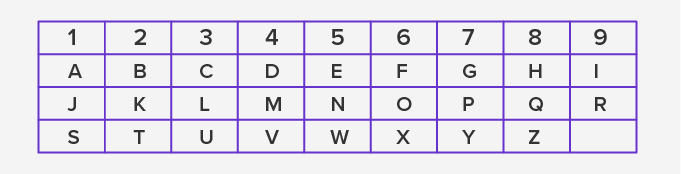# Expression Number - Definition with Examples

The Complete K-5 Math Learning Program Built for Your Child

• 40 Million Kids

Loved by kids and parent worldwide

• 50,000 Schools

Trusted by teachers across schools

• Comprehensive Curriculum

Aligned to Common Core

## Expression Number

Keywords: expression number, expression, numerology, number, master number, single-digit number, core numbers, single-digit

Famous mathematicians believe that the mathematical model is a tool to understand the universe. From the number of elements to the number of stars, we attribute numbers to explain the patterns that exist in the universe.

Scientists and mathematicians use math expressions to establish and prove such relationships.

The concept of expression number uses the technique of assignment of single-digit numbers (1 to 9) to the English alphabet from A to Z. The next section highlights the key developments in mathematics using such number patterns.

## Calculating Expression NumberUsing the above chart, the master number for a name, term or date is evaluated. The single-digit reduction of a given date is the sum of all the numbers until it reaches to a number less than 9.

Considering the name “Tesla” as an example, the expression number will be:

= Tesla = 2 + 5 + 1 + 3 + 1

= 12

= 1 + 2

= 3

For example, consider the date, 10/06/1982. The single digit reduction will be:

= 10/06/1982

= 1 + 0 + 0 + 6 + 1 + 9 + 8 +2

= 27

= 2 + 7

= 9

Thus, the expression number for the given date is 9.

## Understanding digit sum

The concept of digit sum arithmetic is based on expression number. The divisibility properties of numbers are proven using digit sum arithmetic. It is also useful in checking the divisibility of numbers by 3 and 9.

For example, to test whether the number 216 is divisible by 3 and 9, let’s find the digit sum first as follows.

= 216

= 2 + 1 + 6

= 9

= 9 ÷ 3  = 3 with remainder 0

= Also, 216 ÷ 9 = 24 with remainder 0

Thus, the number is divisible by 3 and 9.

## Application

“Modular 9” arithmetic uses the concept of reduction of numbers to single-digit numbers (as digit sum) for studying math axioms. Several statistical models and tests use math expressions to study the behavior of quantitative data from fields like agriculture, finance, economics, and banking. In computer science, the digit sum of binary (base-2) numbers is used to evaluate Hamming weight.

 Fun Facts Economist Edgeworth in the year 1888 used the sum of logarithms to study random number generation and Gaussian distribution. In recreational mathematics, Harshad numbers use the divisibility of the number by the sum of digits.

## Related Math Vocabulary

• Expression

• Arithmetic operators

• Rational expression

• Master number

Won Numerous Awards & Honors